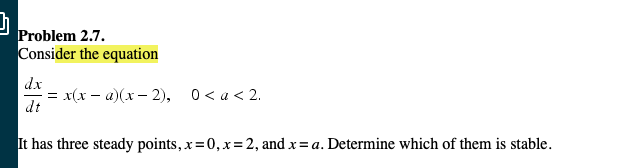# Problem 2.7onsider the equationdx-x(x-u)(x-2),dt0 < a < 2.has three steady points, x=0,x= 2, and x = a. Determine which of them is stable.

Question
37 views

solve problem 2.7help_outlineImage TranscriptioncloseProblem 2.7 onsider the equation dx -x(x-u)(x-2), dt 0 < a < 2. has three steady points, x=0,x= 2, and x = a. Determine which of them is stable. fullscreen
check_circle

Step 1

We have to find stablity of steady point .
Steady  point where dx/dt=0 ,so x=0,x=a,x=2

Step 2

For stability point first we will draw graph between dx/dt and x and check where dx/dt is +ve and -ve.
process are shown in step2 and step 3.

Step 3

With the help of graph we find wh...

### Want to see the full answer?

See Solution

#### Want to see this answer and more?

Solutions are written by subject experts who are available 24/7. Questions are typically answered within 1 hour.*

See Solution
*Response times may vary by subject and question.
Tagged in

### Functions